# infer

Infer conditional variances of conditional variance models

## Syntax

``V = infer(Mdl,Y)``
``Tbl2 = infer(Mdl,Tbl1)``
``````___ = infer(___,Name,Value)``````
``[___,logL] = infer(___)``

## Description

example

````V = infer(Mdl,Y)` returns a numeric array `V` containing the series of conditional variances from evaluating the fully specified, univariate conditional variance model `Mdl` at the numeric array of response data `Y`. `Mdl` can be a `garch`, `egarch`, or `gjr` model.```

example

````Tbl2 = infer(Mdl,Tbl1)` returns the table or timetable `Tbl2` containing the inferred conditional variances and innovations from evaluating the fully specified, univariate conditional variance model `Mdl` at the response variable data in the table or timetable `Tbl1`. When `Mdl` is a model fitted to the response data and returned by `estimate`, the inferred innovations are residuals. (since R2023a)`infer` selects the response variable named in `Mdl.SeriesName` or the sole variable in `Tbl1`. To select a different response variable in `Tbl1` at which to evaluate the model, use the `ResponseVariable` name-value argument.```

example

``````___ = infer(___,Name,Value)``` specifies options using one or more name-value arguments in addition to any of the input argument combinations in previous syntaxes. `infer` returns the output argument combination for the corresponding input arguments. For example, `infer(Mdl,Y,V0=v0)` initializes the conditional variance model of `Mdl` using the presample conditional variance data in `v0`.```

example

````[___,logL] = infer(___)` also returns the loglikelihood objective function values `logL` associated with each inferred path.```

## Examples

collapse all

Infer conditional variances from a GARCH(1,1) model with known coefficients. Specify response data as a numeric vector.

Specify a GARCH(1,1) model with known parameters. Simulate 101 conditional variances and responses (innovations) from the model. Set aside the first observation from each series to use as presample data.

```Mdl = garch(Constant=0.01,GARCH=0.8,ARCH=0.15); rng("default") % For reproducibility [vS,yS] = simulate(Mdl,101); y0 = yS(1); v0 = vS(1); y = yS(2:end); v = vS(2:end); figure tiledlayout(2,1) nexttile plot(v) title("Conditional Variances") nexttile plot(y) title("Innovations") ```Infer the conditional variances of `y` without using presample data. Compare them to the known (simulated) conditional variances.

```vI = infer(Mdl,y); figure plot(1:100,v,"r",LineWidth=2) hold on plot(1:100,vI,"k:",LineWidth=1.5) legend("Simulated","Inferred",Location="northeast") title("Inferred Conditional Variances, No Presample") hold off ```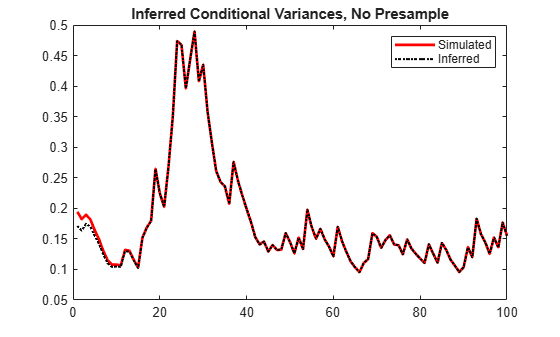Notice the transient response (discrepancy) in the early time periods due to the absence of presample data.

Infer conditional variances using the set-aside presample innovation, `y0`. Compare them to the known (simulated) conditional variances.

```vE = infer(Mdl,y,E0=y0); figure plot(1:100,v,"r",LineWidth=2) hold on plot(1:100,vE,"k:",LineWidth=1.5) legend("Simulated","Inferred",Location="northeast") title("Inferred Conditional Variances, Innovations Presample") hold off ```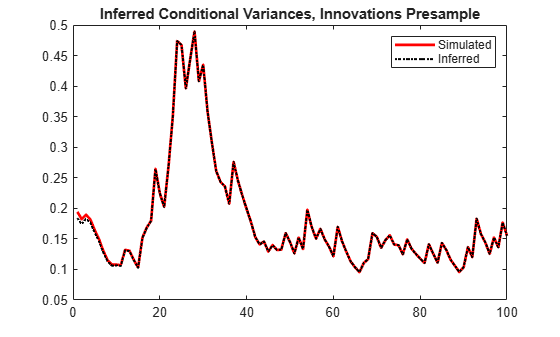There is a slightly reduced transient response in the early time periods.

Infer conditional variances using the presample of conditional variance data, `v0`. Compare them to the known (simulated) conditional variances.

```vO = infer(Mdl,y,V0=v0); figure plot(v) plot(1:100,v,"r",LineWidth=2) hold on plot(1:100,vO,"k:",LineWidth=1.5) legend("Simulated","Inferred",Location="northeast") title("Inferred Conditional Variances, Conditional Variance Presample") hold off ```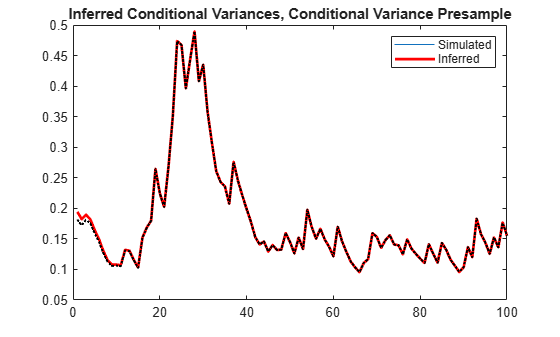There is a much smaller transient response in the early time periods.

Infer conditional variances using both the presample innovation and conditional variance. Compare them to the known (simulated) conditional variances.

```vEO = infer(Mdl,y,E0=y0,V0=v0); figure plot(v) plot(1:100,v,"r",LineWidth=2) hold on plot(1:100,vEO,"k:",LineWidth=1.5) legend("Simulated","Inferred",Location="northeast") title("Inferred Conditional Variances, Presamples") hold off ```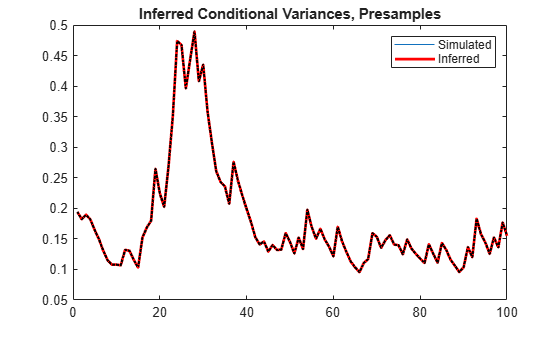When you use sufficient presample innovations and conditional variances, the inferred conditional variances are exact (there is no transient response).

Infer conditional variances from an EGARCH(1,1) model with known coefficients. When you use, and then do not use presample data, compare the results from `infer`.

Specify an EGARCH(1,1) model with known parameters. Simulate 101 conditional variances and responses (innovations) from the model. Set aside the first observation from each series to use as presample data.

```Mdl = egarch(Constant=0.001,GARCH=0.8, ... ARCH=0.15,Leverage=-0.1); rng("default") % For reproducibility [vS,yS] = simulate(Mdl,101); y0 = yS(1); v0 = vS(1); y = yS(2:end); v = vS(2:end); figure tiledlayout(2,1) nexttile plot(v) title("Conditional Variances") nexttile plot(y) title("Innovations") ```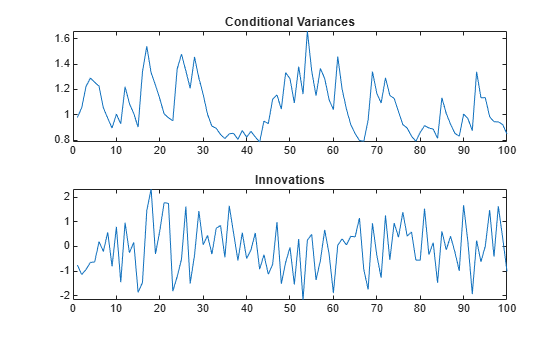Infer the conditional variances of `y` without using any presample data. Compare them to the known (simulated) conditional variances.

```vI = infer(Mdl,y); figure plot(1:100,v,"r",LineWidth=2) hold on plot(1:100,vI,"k:",LineWidth=1.5) legend("Simulated","Inferred",Location="northeast") title("Inferred Conditional Variances, No Presample") hold off ```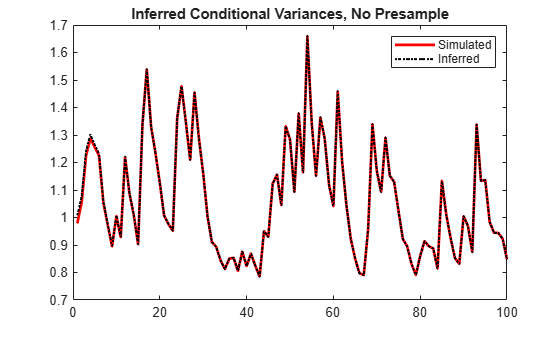Notice the transient response (discrepancy) in the early time periods due to the absence of presample data.

Infer conditional variances using the set-aside presample innovation, `y0`. Compare them to the known (simulated) conditional variances.

```vE = infer(Mdl,y,E0=y0); figure plot(1:100,v,"r",LineWidth=2) hold on plot(1:100,vE,"k:",LineWidth=1.5) legend("Simulated","Inferred",Location="northeast") title("Inferred Conditional Variances, Presample E") hold off ```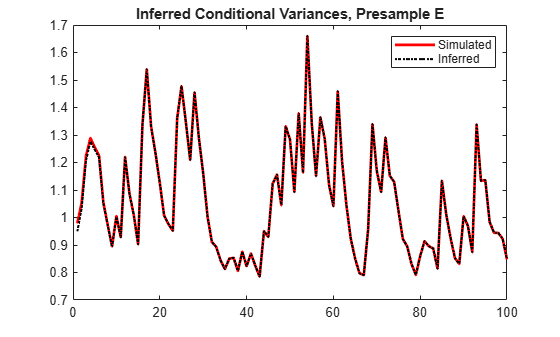There is a slightly reduced transient response in the early time periods.

Infer conditional variances using the set-aside presample variance, `v0`. Compare them to the known (simulated) conditional variances.

```vO = infer(Mdl,y,V0=v0); figure plot(v) plot(1:100,v,"r",LineWidth=2) hold on plot(1:100,vO,"k:",LineWidth=1.5) legend("Simulated","Inferred",Location="northeast") title("Inferred Conditional Variances, Presample V") hold off ```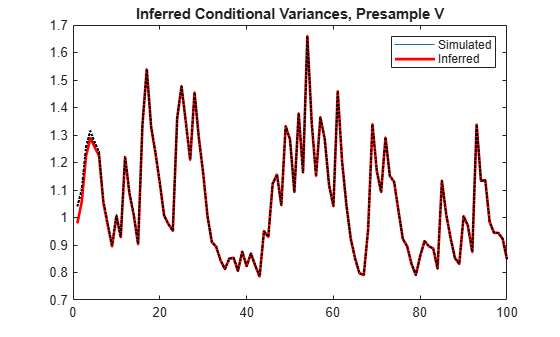The transient response is almost eliminated.

Infer conditional variances using both the presample innovation and conditional variance. Compare them to the known (simulated) conditional variances.

```vEO = infer(Mdl,y,E0=y0,V0=v0); figure plot(v) plot(1:100,v,"r",LineWidth=2) hold on plot(1:100,vEO,"k:",LineWidth=1.5) legend("Simulated","Inferred",Location="northeast") title("Inferred Conditional Variances, Presamples") hold off ```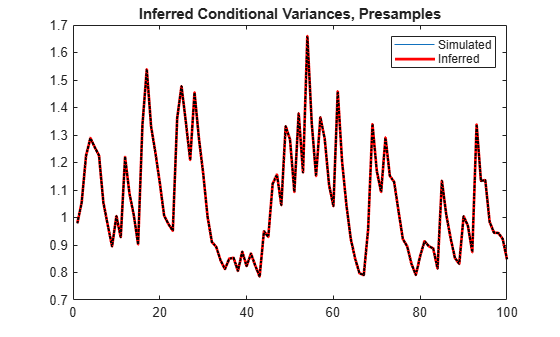When you use sufficient presample innovations and conditional variances, the inferred conditional variances are exact (there is no transient response).

Infer conditional variances from a GJR(1,1) model with known coefficients. When you use, and then do not use presample data, compare the results from `infer`.

Specify a GJR(1,1) model with known parameters. Simulate 101 conditional variances and responses (innovations) from the model. Set aside the first observation from each series to use as presample data.

```Mdl = gjr(Constant=0.01,GARCH=0.8,ARCH=0.14, ... Leverage=0.1); rng("default") % For reproducibility [vS,yS] = simulate(Mdl,101); y0 = yS(1); v0 = vS(1); y = yS(2:end); v = vS(2:end); figure tiledlayout(2,1) nexttile plot(v) title("Conditional Variances") nexttile plot(y) title("Innovations") ```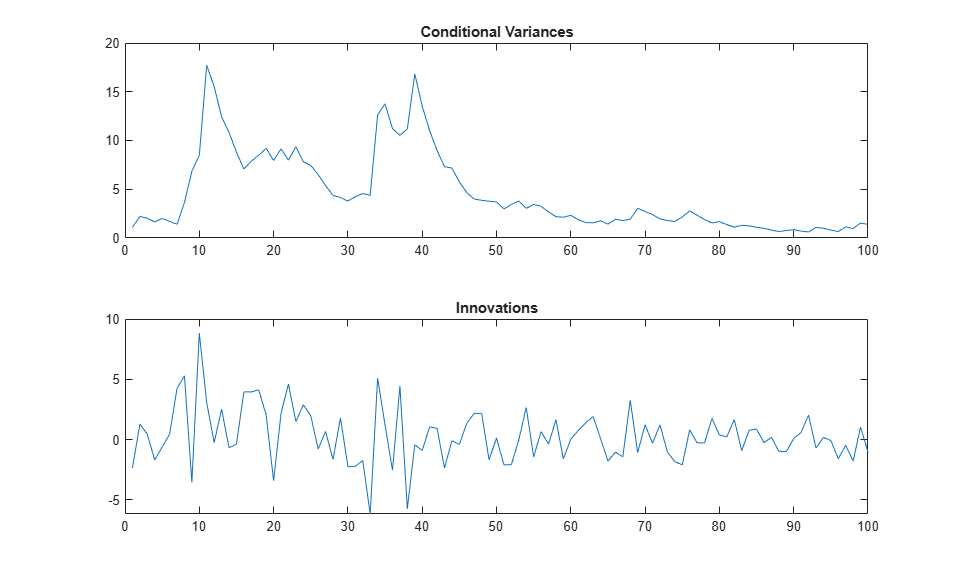Infer the conditional variances of `y` without using any presample data. Compare them to the known (simulated) conditional variances.

```vI = infer(Mdl,y); figure plot(1:100,v,"r",LineWidth=2) hold on plot(1:100,vI,"k:",LineWidth=1.5) legend("Simulated","Inferred",Location="northeast") title("Inferred Conditional Variances, No Presample") hold off ```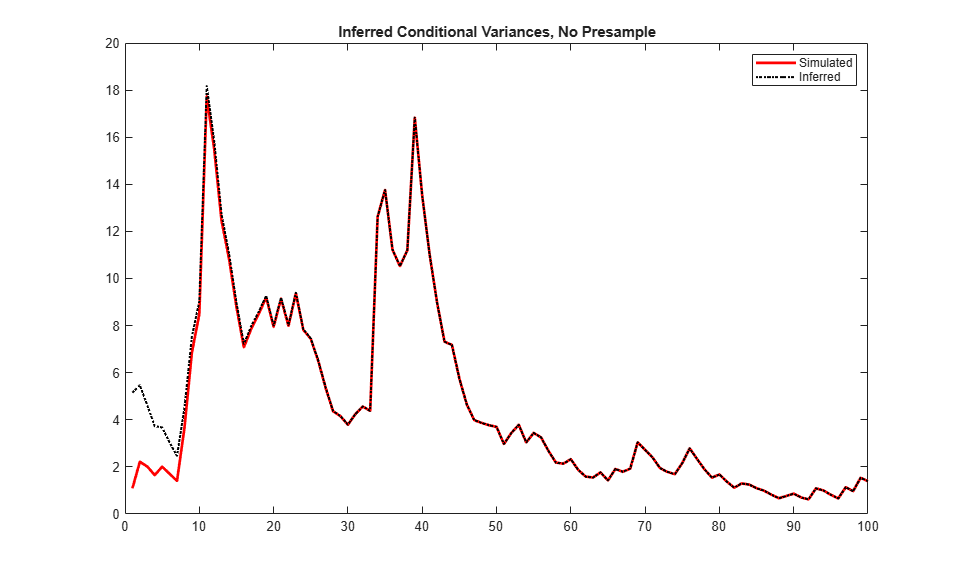Notice the transient response (discrepancy) in the early time periods due to the absence of presample data.

Infer conditional variances using the set-aside presample innovation, `y0`. Compare them to the known (simulated) conditional variances.

```vE = infer(Mdl,y,E0=y0); figure plot(1:100,v,"r",LineWidth=2) hold on plot(1:100,vE,"k:",LineWidth=1.5) legend("Simulated","Inferred",Location="northeast") title("Inferred Conditional Variances, Presample E") hold off ```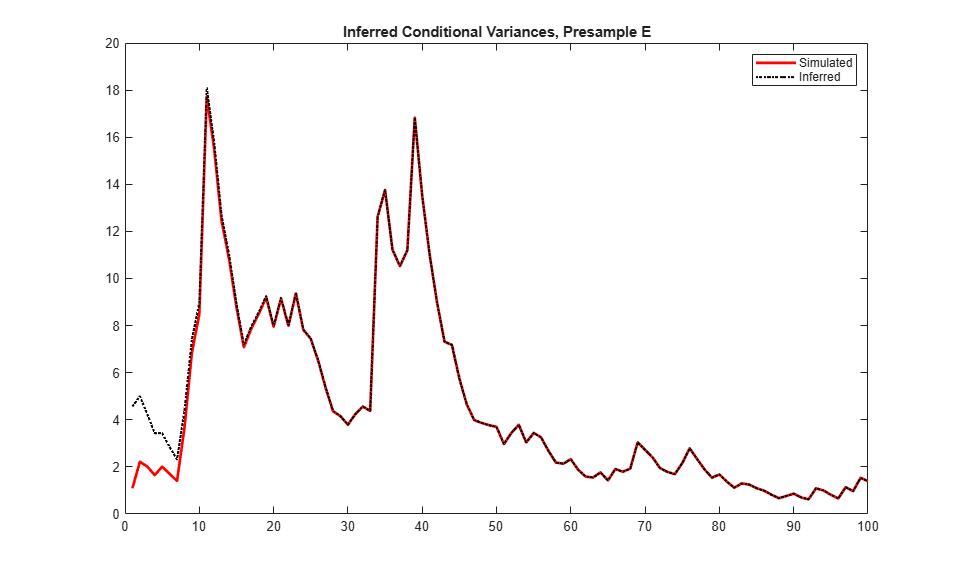There is a slightly reduced transient response in the early time periods.

Infer conditional variances using the set-aside presample conditional variance, `vO`. Compare them to the known (simulated) conditional variances.

```vO = infer(Mdl,y,V0=v0); figure plot(v) plot(1:100,v,"r",LineWidth=2) hold on plot(1:100,vO,"k:",LineWidth=1.5) legend("Simulated","Inferred",Location="northeast") title("Inferred Conditional Variances, Presample V") hold off ```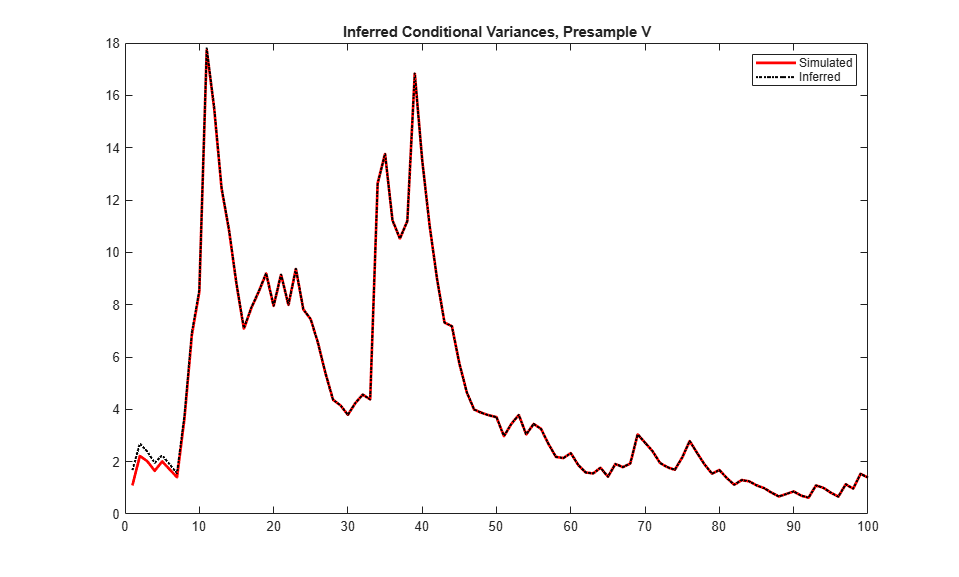There is a much smaller transient response in the early time periods.

Infer conditional variances using both the presample innovation and conditional variance. Compare them to the known (simulated) conditional variances.

```vEO = infer(Mdl,y,E0=y0,V0=v0); figure plot(v) plot(1:100,v,"r",LineWidth=2) hold on plot(1:100,vEO,"k:",LineWidth=1.5) legend("Simulated","Inferred",Location="northeast") title("Inferred Conditional Variances, Presamples") hold off ```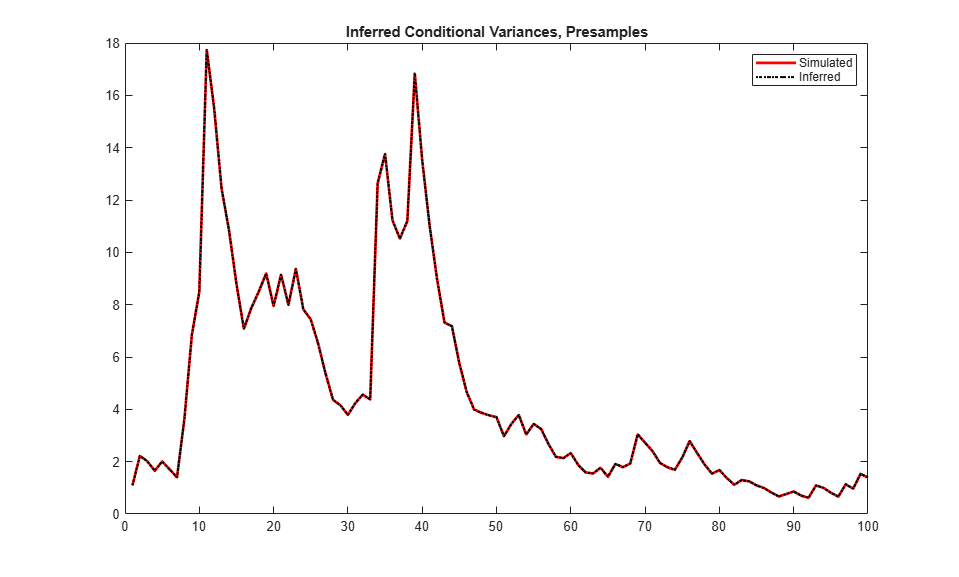When you use sufficient presample innovations and conditional variances, the inferred conditional variances are exact (there is no transient response).

Since R2023a

Infer the loglikelihood objective function values for an EGARCH(1,1) and EGARCH(2,1) model fit to NASDAQ Composite Index returns. To identify which model is the more parsimonious, adequate fit, conduct a likelihood ratio test. Specify data in timetables.

Load the equity index data `Data_EquityIdx`. Convert the index to returns. Reserve the first two observations to use as a presample.

```load Data_EquityIdx DTTRet = price2ret(DataTimeTable); DTTRet.Interval = []; DTTRet0 = DTTRet(1:2,:); DTTRet = DTTRet(3:end,:);```

Fit an EGARCH(1,1) model to the returns. Supply in-sample and presample data in timetables, and specify `NASDAQ` as the variable containing the presample innovations. Infer the loglikelihood objective function value.

```MdlEGARCH11 = egarch(1,1); MdlEGARCH11.SeriesName = "NASDAQ"; EstMdlEGARCH11 = estimate(MdlEGARCH11,DTTRet, ... Presample=DTTRet0,PresampleInnovationVariable="NASDAQ");```
``` EGARCH(1,1) Conditional Variance Model (Gaussian Distribution): Value StandardError TStatistic PValue _________ _____________ __________ __________ Constant -0.13518 0.022134 -6.1073 1.013e-09 GARCH{1} 0.98386 0.0024268 405.41 0 ARCH{1} 0.19997 0.013993 14.29 2.5182e-46 Leverage{1} -0.060244 0.0056558 -10.652 1.7131e-26 ```
```[TblEGARCH11,logLEGARCH11] = infer(EstMdlEGARCH11,DTTRet, ... Presample=DTTRet0,PresampleInnovationVariable="NASDAQ"); tail(TblEGARCH11)```
``` Time NYSE NASDAQ NASDAQ_Variance NASDAQ_Residual ___________ __________ ___________ _______________ _______________ 10-Apr-1998 0.0078238 -0.010969 0.00029328 -0.010969 11-Apr-1998 -0.0031839 -0.032991 0.00029422 -0.032991 12-Apr-1998 0.0041747 0.014124 0.0004121 0.014124 13-Apr-1998 0.0005974 -0.00069403 0.00038357 -0.00069403 14-Apr-1998 0.0043927 0.008307 0.00032732 0.008307 15-Apr-1998 0.005844 0.0079856 0.00029585 0.0079856 16-Apr-1998 0.0038771 0.0054697 0.00026803 0.0054697 17-Apr-1998 -0.0077353 -0.018722 0.00023883 -0.018722 ```

`TblEGARCH11` is a timetable of NASDAQ residuals `NASDAQ_Residual` and conditional variances `NASDAQ_Variance`, and all variables in the specified in-sample data `DTTRet`. `logLEGARCH11` is the loglikelihood of the estimated model `EstMdlEGARCH11` evaluated at the specified presample and in-sample data.

Fit an EGARCH(2,1) model to the returns. Supply in-sample and presample data in timetables, and specify `NASDAQ` as the variable containing the presample innovations. Infer the loglikelihood objective function value.

```MdlEGARCH21 = egarch(2,1); MdlEGARCH21.SeriesName = "NASDAQ"; EstMdlEGARCH21 = estimate(MdlEGARCH21,DTTRet, ... Presample=DTTRet0,PresampleInnovationVariable="NASDAQ");```
``` EGARCH(2,1) Conditional Variance Model (Gaussian Distribution): Value StandardError TStatistic PValue _________ _____________ __________ __________ Constant -0.1456 0.028436 -5.1202 3.0526e-07 GARCH{1} 0.85307 0.14018 6.0854 1.1618e-09 GARCH{2} 0.12952 0.13838 0.93599 0.34928 ARCH{1} 0.21969 0.029465 7.456 8.9198e-14 Leverage{1} -0.067936 0.010879 -6.2444 4.255e-10 ```
```[TblEGARCH21,logLEGARCH21] = infer(EstMdlEGARCH21,DTTRet, ... Presample=DTTRet0,PresampleInnovationVariable="NASDAQ");```

Conduct a likelihood ratio test, with the more parsimonious EGARCH(1,1) model as the null model, and the EGARCH(2,1) model as the alternative. The degree of freedom for the test is 1, because the EGARCH(2,1) model has one more parameter than the EGARCH(1,1) model (an additional GARCH term).

`[h,p] = lratiotest(logLEGARCH21,logLEGARCH11,1)`
```h = logical 0 ```
```p = 0.2256 ```

The null hypothesis is not rejected (`h = 0`). At the 0.05 significance level, the EGARCH(1,1) model is not rejected in favor of the EGARCH(2,1) model.

A GARCH(P, Q) model is nested within a GJR(P, Q) model. Therefore, you can perform a likelihood ratio test to compare GARCH(P, Q) and GJR(P, Q) model fits.

Infer the loglikelihood objective function values for a GARCH(1,1) and GJR(1,1) model fit to NASDAQ Composite Index returns. Conduct a likelihood ratio test to identify which model is the more parsimonious, adequate fit.

Load the NASDAQ data included with the toolbox, and convert the index to returns. Set aside the first two observations to use as presample data.

```load Data_EquityIdx nasdaq = DataTable.NASDAQ; r = price2ret(nasdaq); r0 = r(1:2); rn = r(3:end);```

Fit a GARCH(1,1) model to the returns, and infer the loglikelihood objective function value.

```Mdl1 = garch(1,1); EstMdl1 = estimate(Mdl1,rn,E0=r0);```
``` GARCH(1,1) Conditional Variance Model (Gaussian Distribution): Value StandardError TStatistic PValue _________ _____________ __________ __________ Constant 2.005e-06 5.4298e-07 3.6926 0.00022197 GARCH{1} 0.88333 0.0084536 104.49 0 ARCH{1} 0.10924 0.0076666 14.249 4.5739e-46 ```
`[~,logL1] = infer(EstMdl1,rn,E0=r0);`

Fit a GJR(1,1) model to the returns, and infer the loglikelihood objective function value.

```Mdl2 = gjr(1,1); EstMdl2 = estimate(Mdl2,rn,E0=r0);```
``` GJR(1,1) Conditional Variance Model (Gaussian Distribution): Value StandardError TStatistic PValue __________ _____________ __________ __________ Constant 2.4754e-06 5.6986e-07 4.3439 1.3997e-05 GARCH{1} 0.88101 0.0095108 92.632 0 ARCH{1} 0.064017 0.0091852 6.9696 3.1794e-12 Leverage{1} 0.089301 0.0099215 9.0007 2.2429e-19 ```
`[~,logL2] = infer(EstMdl2,rn,E0=r0);`

Conduct a likelihood ratio test, with the more parsimonious GARCH(1,1) model as the null model, and the GJR(1,1) model as the alternative. The degree of freedom for the test is 1, because the GJR(1,1) model has one more parameter than the GARCH(1,1) model (a leverage term).

`[h,p] = lratiotest(logL2,logL1,1)`
```h = logical 1 ```
```p = 4.5815e-10 ```

The null hypothesis is rejected (`h = 1`). At the 0.05 significance level, the GARCH(1,1) model is rejected in favor of the GJR(1,1) model.

## Input Arguments

collapse all

Conditional variance model without any unknown parameters, specified as a `garch`, `egarch`, or `gjr` model object.

`Mdl` cannot contain any properties that have `NaN` value.

Response data, specified as a `numobs`-by-1 numeric column vector or `numobs`-by-`numpaths` matrix.

As a column vector, `Y` represents a single path of the underlying series.

As a matrix, the rows of `Y` correspond to periods and the columns correspond to separate paths. The observations across any row occur simultaneously.

`infer` infers the conditional variances of `Y`. `Y` usually represents an innovation series with mean 0 and variances characterized by `Mdl`. It is the continuation of the presample innovation series `E0`. `Y` can also represent a time series of innovations with mean 0 plus an offset. If `Mdl` has a nonzero offset, then the software stores its value in the `Offset` property (`Mdl.Offset`).

The last observation of any series is the latest observation.

Since R2023a

Time series data containing response variable yt, at which `infer` evaluates the conditional variance model `Mdl`, specified as a table or timetable with `numvars` variables and `numobs` rows. You can optionally select a response variable by using the `ResponseVariable` name-value argument.

The selected variable is a single path (`numobs`-by-1 vector) or multiple paths (`numobs`-by-`numpaths` matrix) of `numobs` observations of response data. Each row is an observation, and measurements in each row occur simultaneously.

The selected response variable in `Tbl1` is a `numobs`-by-`numpaths` numeric matrix. Each row is an observation, and measurements in each row occur simultaneously.

Each path (column) of the selected variable is independent of the other paths.

If `Tbl1` is a timetable, it must represent a sample with a regular datetime time step (see `isregular`), and the datetime vector `Tbl1.Time` must be strictly ascending or descending.

If `Tbl1` is a table, the last row contains the latest observation.

### Name-Value Arguments

Specify optional pairs of arguments as `Name1=Value1,...,NameN=ValueN`, where `Name` is the argument name and `Value` is the corresponding value. Name-value arguments must appear after other arguments, but the order of the pairs does not matter.

Before R2021a, use commas to separate each name and value, and enclose `Name` in quotes.

Example: `'E0',[1 1;0.5 0.5],'V0',[1 0.5;1 0.5]` specifies two equivalent presample paths of innovations and two, different presample paths of conditional variances.

Since R2023a

Variable to select from `Tbl1` to treat as the response variable yt, specified as one of the following data types:

• String scalar or character vector containing a variable name in `Tbl1.Properties.VariableNames`

• Variable index (integer) to select from `Tbl1.Properties.VariableNames`

• A length `numvars` logical vector, where ```ResponseVariable(j) = true``` selects variable `j` from `Tbl1.Properties.VariableNames`, and `sum(ResponseVariable)` is `1`

The selected variable must be a numeric vector and cannot contain missing values (`NaN`).

If `Tbl1` has one variable, the default specifies that variable. Otherwise, the default matches the variable to name in `Mdl.SeriesName`.

Example: `ResponseVariable="StockRate2"`

Example: `ResponseVariable=[false false true false]` or `ResponseVariable=3` selects the third table variable as the response variable.

Data Types: `double` | `logical` | `char` | `cell` | `string`

Presample innovation paths εt, specified as a `numpreobs`-by-1 numeric column vector or a `numpreobs`-by-`numprepaths` matrix. The presample innovations provide initial values for the innovations process of the conditional variance model `Mdl`, and derive from a distribution with mean 0. Use `E0` only when you supply the numeric array of response data `Y`.

`numpreobs` is the number of presample observations. `numprepaths` is the number of presample response paths.

Each row is a presample observation, and measurements in each row occur simultaneously. The last row contains the latest presample observation. `numpreobs` must be at least `Mdl.Q`. If `numpreobs` > `Mdl.Q`, `infer` uses the latest required number of observations only. The last element or row contains the latest observation.

• If `E0` is a column vector, it represents a single path of the underlying innovation series. `infer` applies it to each output path.

• If `E0` is a matrix, each column represents a presample path of the underlying innovation series. `numprepaths` must be at least `numpaths`. If `numprepaths` > `numpaths`, `infer` uses the first `size(Y,2)` columns only.

The defaults are:

• For GARCH(P,Q) and GJR(P,Q) models, `infer` sets any necessary presample innovations to the square root of the average squared value of the offset-adjusted response series `Y`.

• For EGARCH(P,Q) models, `infer` sets any necessary presample innovations to zero.

Data Types: `double`

Positive presample conditional variance paths σt2, specified as a `numpreobs`-by-1 positive column vector or `numpreobs`-by-`numprepaths` positive matrix. `V0` provides initial values for the conditional variances in the model. Use `V0` only when you supply the numeric array of disturbances `Z`.

Each row is a presample observation, and measurements in each row occur simultaneously. The last row contains the latest presample observation.

• For GARCH(P,Q) and GJR(P,Q) models, `numpreobs` must be at least `Mdl.P`.

• For EGARCH(P,Q) models,`numpreobs` must be at least `max([Mdl.P Mdl.Q])`.

`numpreobs` must be at least `max([Mdl.P Mdl.Q])`. If `numpreobs` > ```max([Mdl.P Mdl.Q])```, `infer` uses the latest required number of observations only. The last element or row contains the latest observation.

• If `V0` is a column vector, it represents a single path of the conditional variance series. `infer` applies it to each output path.

• If `V0` is a matrix, each column represents a presample path of the conditional variance series. `numprepaths` must be at least `numpaths`. If `numprepaths` > `numpaths`, `infer` uses the first `size(Y,2)` columns only.

By default, `infer` sets any necessary presample conditional variances to the unconditional variance of the process.

Data Types: `double`

Since R2023a

Presample data for innovations εt or conditional variances σt2 to initialize the model, specified as a table or timetable, the same type as `Tbl1`, with `numprevars` variables and `numpreobs` rows. Use `Presample` only when you supply a table or timetable of data `Tbl1`.

Each selected variable is a single path (`numpreobs`-by-1 vector) or multiple paths (`numpreobs`-by-`numprepaths` matrix) of `numpreobs` observations representing the presample of `numpreobs` observations of innovations or conditional variances for `ResponseVariable`, the selected response variable in `Tbl1`.

Each row is a presample observation, and measurements in each row occur simultaneously. `numpreobs` must be one of the following values:

• `Mdl.Q` when `Presample` provides only presample innovations.

• `Mdl.P` when `Presample` provides only presample conditional variances.

• `max([Mdl.P Mdl.Q])` when `Presample` provides both presample innovations and conditional variances

If `numpreobs` exceeds the minimum number, `infer` uses the latest required number of observations only.

If `Presample` is a timetable, all the following conditions must be true:

• `Presample` must represent a sample with a regular datetime time step (see `isregular`).

• The inputs `Tbl1` and `Presample` must be consistent in time such that `Presample` immediately precedes `Tbl1` with respect to the sampling frequency and order.

• The datetime vector of sample timestamps `Presample.Time` must be ascending or descending.

If `Presample` is a table, the last row contains the latest presample observation.

The defaults are:

• For GARCH(P,Q) and GJR(P,Q) models, `infer` sets any necessary presample innovations to the square root of the average squared value of the offset-adjusted response series `Y`.

• For EGARCH(P,Q) models, `infer` sets any necessary presample innovations to zero.

• `infer` sets any necessary presample conditional variances to the unconditional variance of the process.

If you specify the `Presample`, you must specify the presample innovation or conditional variance variable names by using the `PresampleInnovationVariable` or `PresampleVarianceVariable` name-value argument.

Since R2023a

Variable of `Presample` containing presample innovation paths εt, specified as one of the following data types:

• String scalar or character vector containing a variable name in `Presample.Properties.VariableNames`

• Variable index (integer) to select from `Presample.Properties.VariableNames`

• A length `numprevars` logical vector, where `PresampleInnovationVariable(j) = true` selects variable `j` from `Presample.Properties.VariableNames`, and `sum(PresampleInnovationVariable)` is `1`

The selected variable must be a numeric matrix and cannot contain missing values (`NaN`).

If you specify presample innovation data by using the `Presample` name-value argument, you must specify `PresampleInnovationVariable`.

Example: `PresampleInnovationVariable="StockRateInnov0"`

Example: `PresampleInnovationVariable=[false false true false]` or `PresampleInnovationVariable=3` selects the third table variable as the presample innovation variable.

Data Types: `double` | `logical` | `char` | `cell` | `string`

Since R2023a

Variable of `Presample` containing data for the presample conditional variances σt2, specified as one of the following data types:

• String scalar or character vector containing a variable name in `Presample.Properties.VariableNames`

• Variable index (integer) to select from `Presample.Properties.VariableNames`

• A length `numprevars` logical vector, where `PresampleVarianceVariable(j) = true` selects variable `j` from `Presample.Properties.VariableNames`, and `sum(PresampleVarianceVariable)` is `1`

The selected variable must be a numeric vector and cannot contain missing values (`NaN`).

If you specify presample conditional variance data by using the `Presample` name-value argument, you must specify `PresampleVarianceVariable`.

Example: `PresampleVarianceVariable="StockRateVar0"`

Example: `PresampleVarianceVariable=[false false true false]` or `PresampleVarianceVariable=3` selects the third table variable as the presample conditional variance variable.

Data Types: `double` | `logical` | `char` | `cell` | `string`

Notes:

• `NaN` values in `Y`, `E0`, and `V0` indicate missing values. `infer` removes missing values from specified data by list-wise deletion.

• For the presample, `infer` horizontally concatenates `E0` and `V0`, and then it removes any row of the concatenated matrix containing at least one `NaN`.

• For in-sample data `Y`, `infer` removes any row containing at least one `NaN`.

This type of data reduction reduces the effective sample size and can create an irregular time series.

• For numeric data inputs, `infer` assumes that you synchronize the presample data such that the latest observations occur simultaneously.

• `infer` issues an error when any table or timetable input contains missing values.

## Output Arguments

collapse all

Conditional variances inferred from the response data `Y`, returned as a numeric column vector or matrix. `infer` returns `V` only when you supply the input `Y`.

The dimensions of `V` and `Y` are equivalent. If `Y` is a matrix, then the columns of `V` are the inferred conditional variance paths corresponding to the columns of `Y`.

Rows of `V` are periods corresponding to the periodicity of `Y`.

Loglikelihood objective function values associated with the model `Mdl`, returned as a scalar or numeric vector.

If `Y` is a vector, then `logL` is a scalar. Otherwise, `logL` is vector of length `size(Y,2)`, and each element is the loglikelihood of the corresponding column (or path) in `Y`.

Data Types: `double`

Inferred conditional variance σt2 and innovation εt paths, returned as a table or timetable, the same data type as `Tbl1`. `infer` returns `Tbl2` only when you supply the input `Tbl1`. When `Mdl` is an estimated model returned by `estimate`, the returned, inferred innovations are residuals.

`Tbl2` contains the following variables:

• The inferred conditional variance paths, which are in a `numobs`-by-`numpaths` numeric matrix, with rows representing observations and columns representing independent paths. Each path represents the continuation of the corresponding path of presample conditional variances in `Presample`. `infer` names the filtered conditional variance variable in `Tbl2` `responseName_Variance`, where `responseName` is `Mdl.SeriesName`. For example, if `Mdl.SeriesName` is `StockReturns`, `Tbl2` contains a variable for the corresponding inferred conditional variance paths with the name `StockReturns_Variance`.

• The inferred innovation paths, which are in a `numobs`-by-`numpaths` numeric matrix, with rows representing observations and columns representing independent paths. Each path corresponds to the input response path in `Tbl1` and represents the continuation of the corresponding presample innovations path in `Presample`. `infer` names the inferred innovations variable in `Tbl2` `responseName_Residual`, where `responseName` is `Mdl.SeriesName`. For example, if `Mdl.SeriesName` is `StockReturns`, `Tbl2` contains a variable for the corresponding inferred innovations paths with the name `StockReturns_Residual`.

• All variables `Tbl1`.

If `Tbl1` is a timetable, row times of `Tbl1` and `Tbl2` are equal.

## Algorithms

If you do not specify presample data (`E0` and `V0`, or `Presample`), `infer` derives the necessary presample observations from the unconditional, or long-run, variance of the offset-adjusted response process.

• For all conditional variance model types, required presample conditional variances are the sample average of the squared disturbances of the offset-adjusted specified response data (`Y` or `Tbl1`).

• For GARCH(P,Q) and GJR(P,Q) models, the required presample innovations are the square root of the average squared value of the offset-adjusted response data.

• For EGARCH(P,Q) models, the required presample innovaitons are `0`.

These specifications minimize initial transient effects.

 Bollerslev, T. “Generalized Autoregressive Conditional Heteroskedasticity.” Journal of Econometrics. Vol. 31, 1986, pp. 307–327.

 Bollerslev, T. “A Conditionally Heteroskedastic Time Series Model for Speculative Prices and Rates of Return.” The Review of Economics and Statistics. Vol. 69, 1987, pp. 542–547.

 Box, G. E. P., G. M. Jenkins, and G. C. Reinsel. Time Series Analysis: Forecasting and Control. 3rd ed. Englewood Cliffs, NJ: Prentice Hall, 1994.

 Enders, W. Applied Econometric Time Series. Hoboken, NJ: John Wiley & Sons, 1995.

 Engle, R. F. “Autoregressive Conditional Heteroskedasticity with Estimates of the Variance of United Kingdom Inflation.” Econometrica. Vol. 50, 1982, pp. 987–1007.

 Glosten, L. R., R. Jagannathan, and D. E. Runkle. “On the Relation between the Expected Value and the Volatility of the Nominal Excess Return on Stocks.” The Journal of Finance. Vol. 48, No. 5, 1993, pp. 1779–1801.

 Hamilton, J. D. Time Series Analysis. Princeton, NJ: Princeton University Press, 1994.